# How to Calculate the Radius, Height and Curved Surface Area of a Spherical Cap | The Calculator Encyclopedia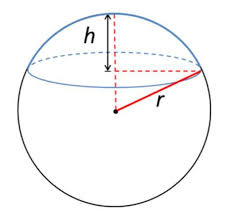The image above is a spherical cap.

To compute the curved surface area of a spherical cap requires two essential parameters which are the radius of the base of the cap (a) and the height (h).

The formula for calculating the curved surface area of the spherical cap:

A = π(a² + h²)

Where;
A = Curved surface area of the spherical cap
a = Radius of the base of the cap
h = Height of the spherical cap

Let’s solve an example;
Find the curved surface area of a spherical cap with radius of the base 7 cm and the height of 13 cm.

This implies that;
a = Radius of the base of the cap = 7 cm
h = Height of the spherical cap = 13 cm

A = π(a² + h²)
A =  π(7² + 13²)
A =  π(49 + 169)
A =  π(218)
A = 684.867

Therefore, the curved surface area of the spherical cap is 684.867 cm².

Calculating the Radius of the base of a Spherical Cap using the Curved Surface Area of the Spherical Cap and the Height.

a = √A – πh2 / π

Where;
A = Curved surface area of the spherical cap
a = Radius of the base of the cap
h = Height of the spherical cap

Let’s solve an example;
Find the radius of the base of a spherical cap when the curved surface area of the spherical cap is 300 cm2 and a height of 7 cm.

This implies that;
A = Curved surface area of the spherical cap = 300 cm2
h = Height of the spherical cap = 7 cm

a = √A – πh2 / π
a = √300 – 3.142 x 72 / π
a = √300 – 3.142 x 49 / π
a = √300 – 153.958 / π
a = √146.042 / π
a = √46.48
a = 6.82

Therefore, the radius of the base of the cap is 6.82 cm.

Calculating the Height of a Spherical Cap using the Curved Surface Area of the Spherical Cap and the Radius of the base of the Cap.

h = √A – πa2 / π

Where;
A = Curved surface area of the spherical cap
a = Radius of the base of the cap
h = Height of the spherical cap

Let’s solve an example;
Find the height of a spherical cap when the curved surface area of the spherical cap is 420 cm2 and the radius of the base of the cap is 9 cm.

This implies that;
A = Curved surface area of the spherical cap = 420 cm2
r = Radius of the base of the spherical cap = 9 cm

h = √A – πa2 / π
h = √420 – 3.142 x 92 / π
h = √420 – 3.142 x 81 / π
h = √420 – 254.502 / π
h = √165.498 / π
h = √52.67
h = 7.26

Therefore, the height of the spherical cap is 7.26 cm.

Nickzom Calculator – The Calculator Encyclopedia is capable of calculating the curved surface area of the spherical cap.

To get the answer and workings of the curved surface area of the spherical cap using the Nickzom Calculator – The Calculator Encyclopedia. First, you need to obtain the app.

You can get this app via any of these means:

To get access to the professional version via web, you need to register and subscribe for NGN 1,500 per annum to have utter access to all functionalities.
You can also try the demo version via https://www.nickzom.org/calculator

Once, you have obtained the calculator encyclopedia app, proceed to the Calculator Map, then click on Mensuration under the Mathematics section.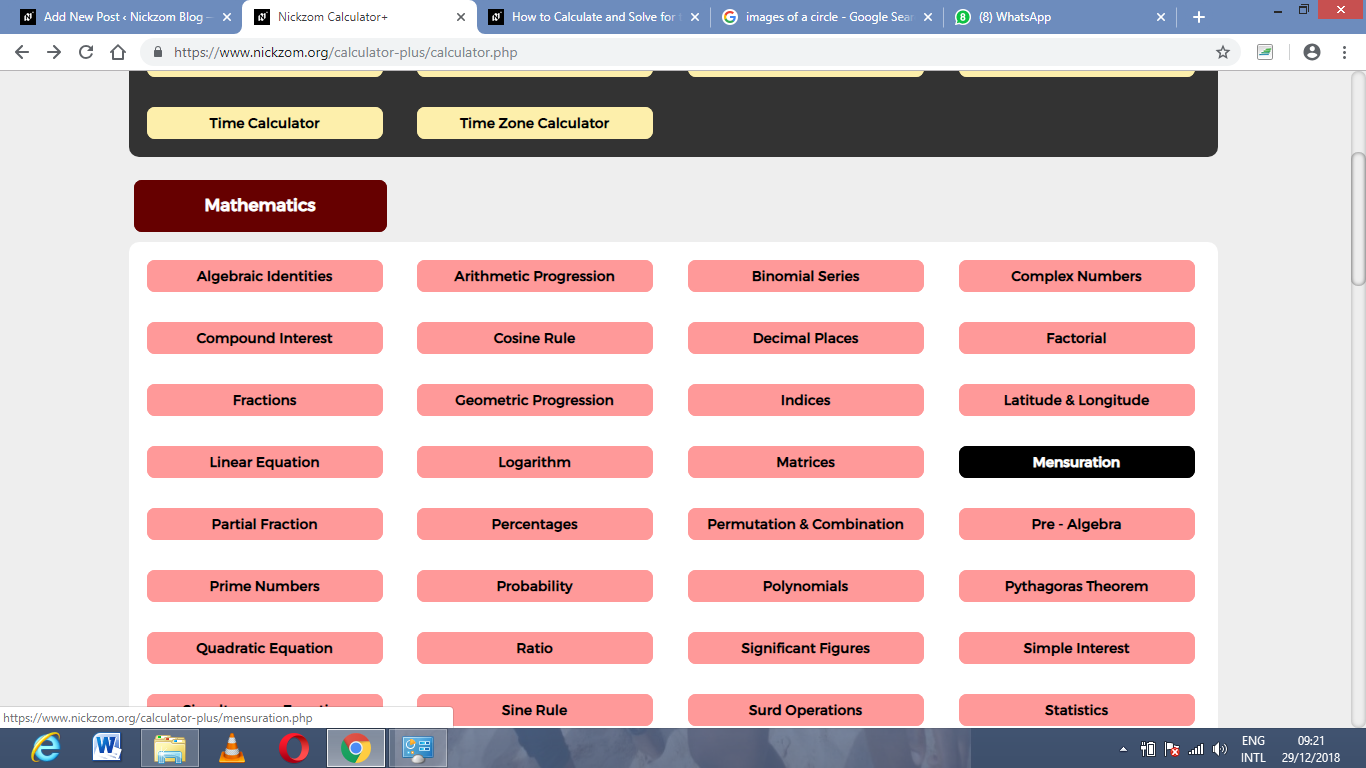Now, click on Curved Surface Area of the spherical cap under Mensuration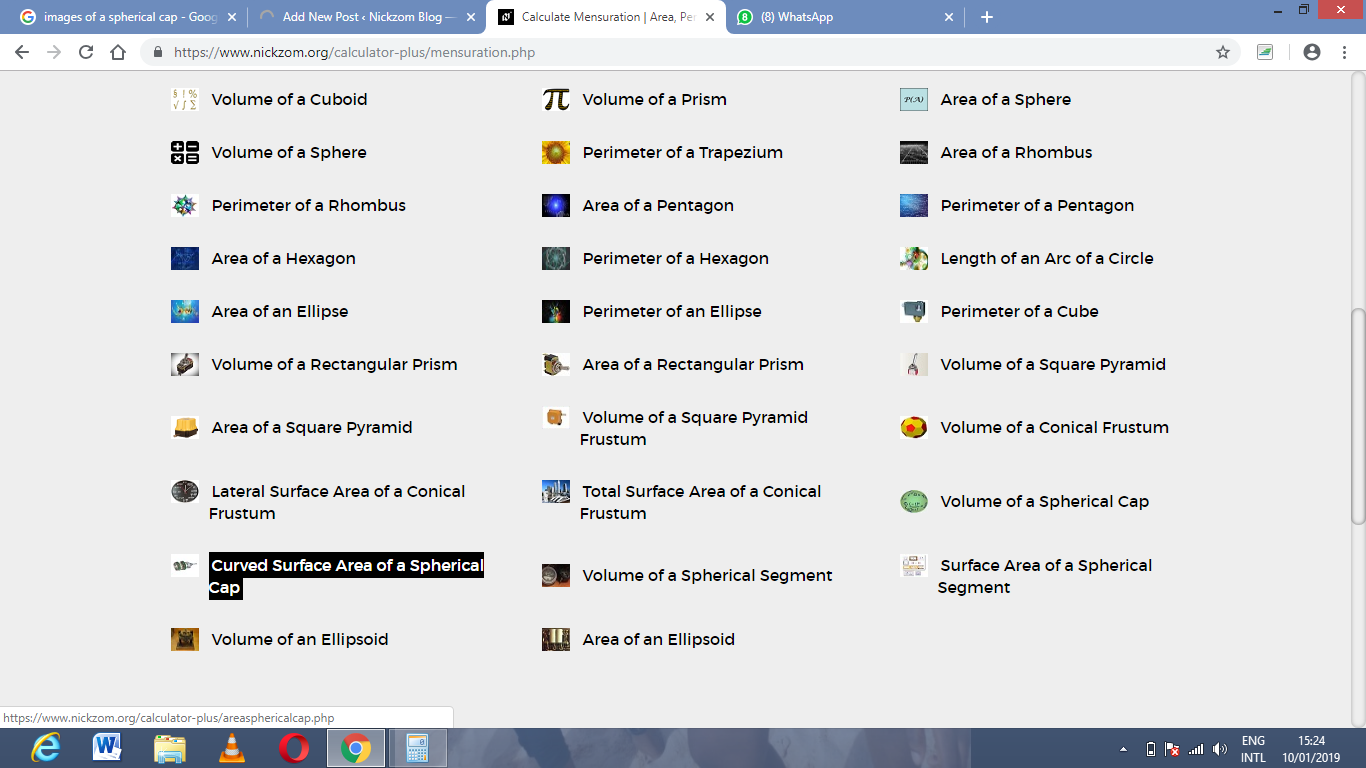The screenshot below displays the page or activity to enter your values, to get the answer for the curved surface area of the spherical cap according to the respective parameters which are the radius of the base of the cap (a) and height (h).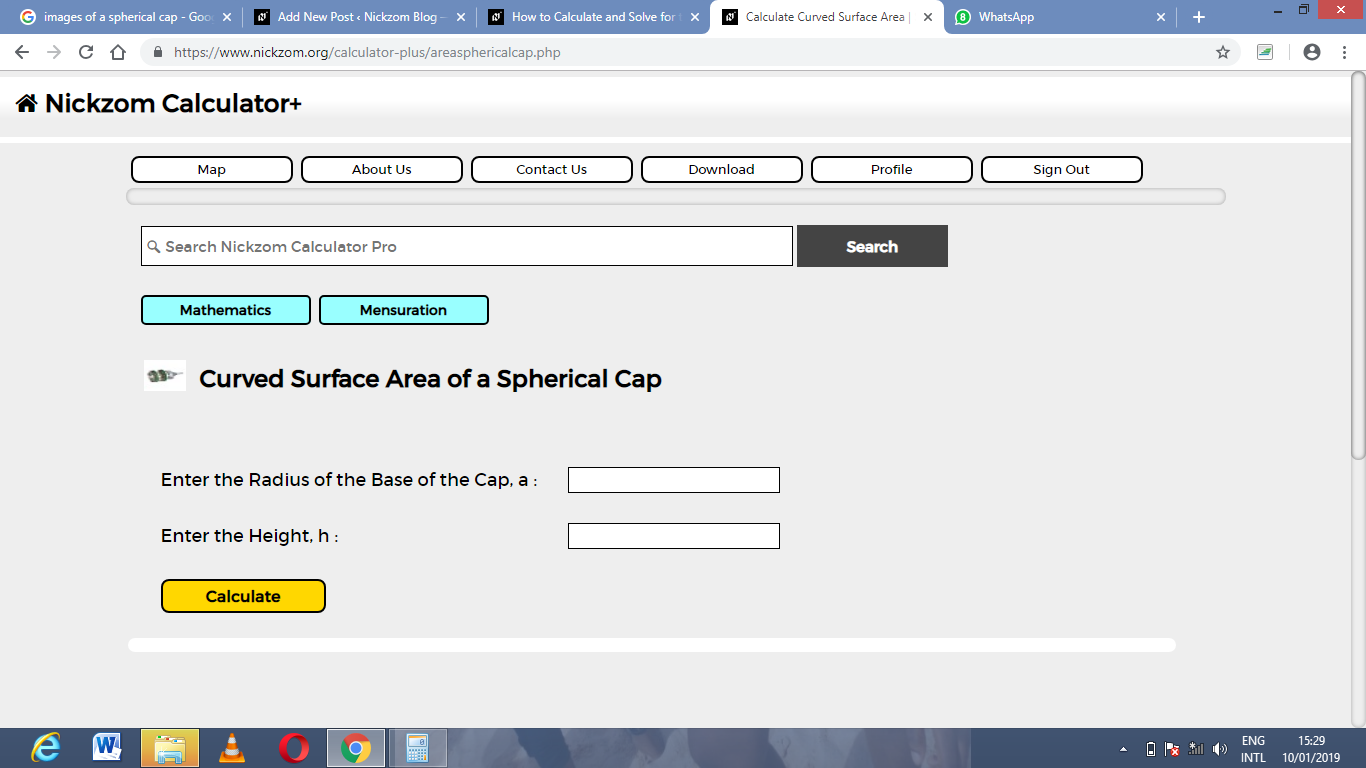Now, enter the values appropriately and accordingly for the parameters as required by the example above where the radius of the base of the cap is 7 cm and the height is 13 cm.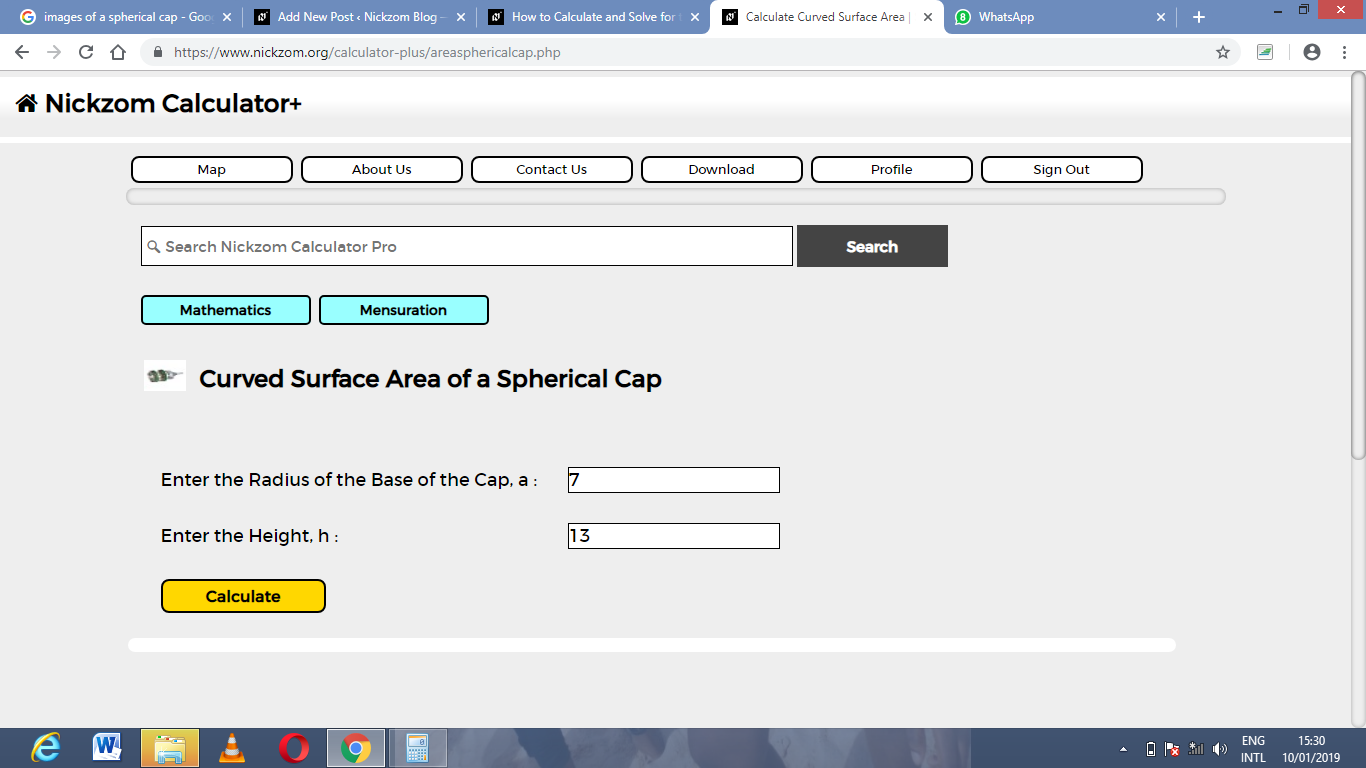Finally, Click on Calculate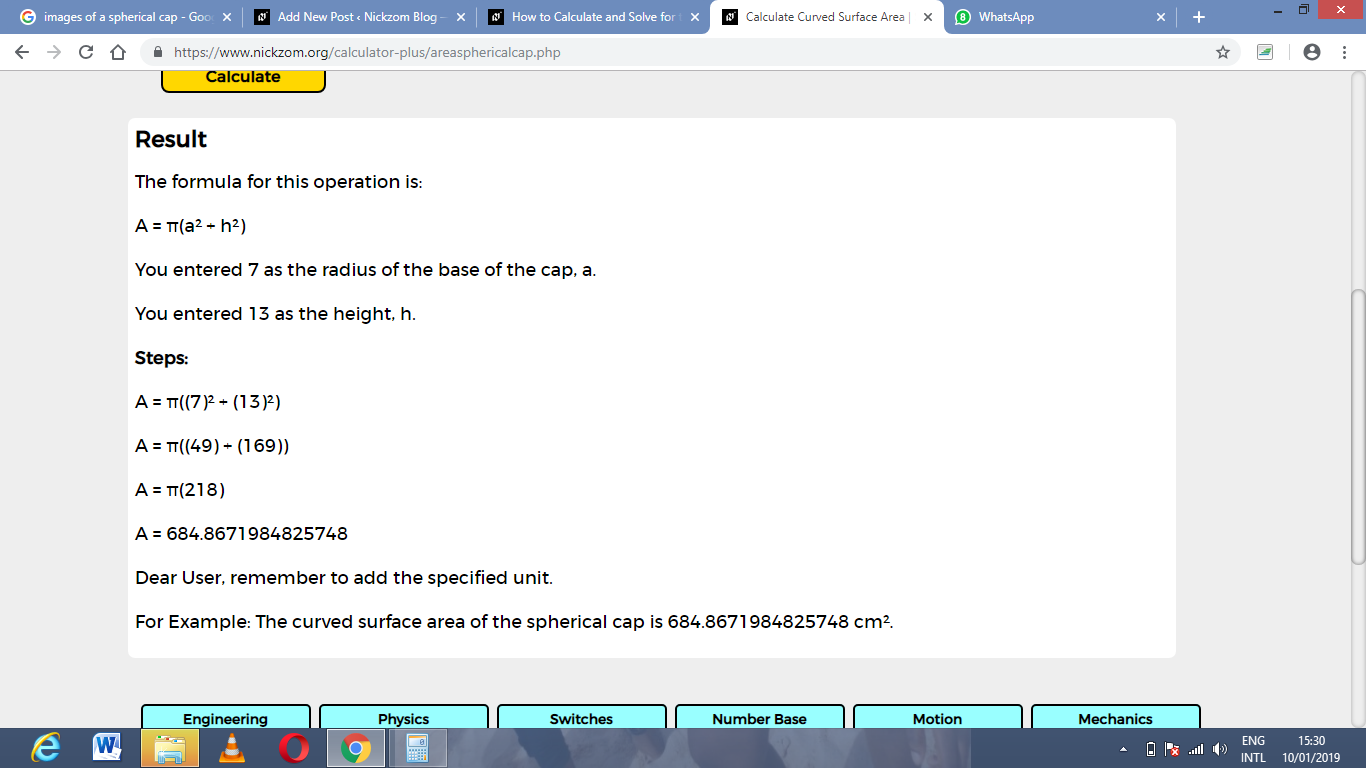As you can see from the screenshot above, Nickzom Calculator – The Calculator Encyclopedia solves for the Curved Surface Area of a spherical cap and presents the formula, workings and steps too.